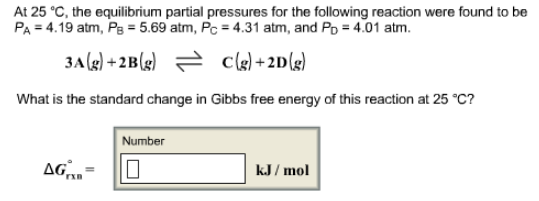# Problem: At 25°C, the equilibrium partial pressures for the following reaction were found to be PA = 4.19 atm, PB = 5.69 atm, PC = 4.31 atrm, and PD = 4.01 atm. 3A (g) + 2B (g) ⇌ C (g) + 2D (g) What is the standard change in Gibbs free energy of this reaction at 25°C?

###### FREE Expert Solution###### Problem Details

At 25°C, the equilibrium partial pressures for the following reaction were found to be PA = 4.19 atm, PB = 5.69 atm, PC = 4.31 atrm, and PD = 4.01 atm.

3A (g) + 2B (g) ⇌ C (g) + 2D (g)

What is the standard change in Gibbs free energy of this reaction at 25°C?